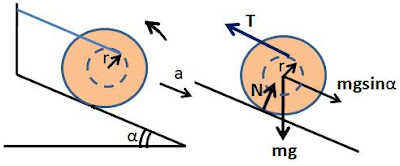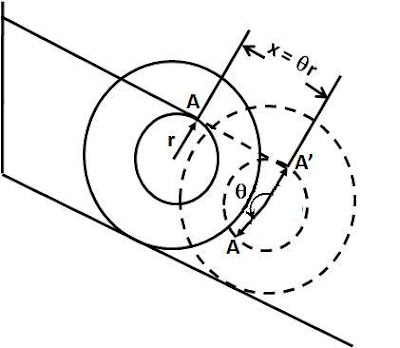Friday, December 12, 2008

Irodov Problem 1.255There are three forces acting on the spindle at any point of time, i) the force of gravity mg acting downwards, ii) the normal reaction from the surface N and iii) the tension in the string T. Since there is no friction between the surface of the plane and the spool, the spool will only slip, it will never roll. Further there will be no force of friction to resist the slipping of the spool downwards. Let the acceleration of the spool be a then considering the forces in the direction of the inclined plane we have,Since both the normal reaction N and the force of gravity mg pass through the axis of rotation of the spool they do not cause any torque on the spool. However, the tension in the string acts at a distance r from the axis and so it will induce a torque Tr on the spool and cause it to spin. Let the angular acceleration of the spool be, then we have,Now the only way for the spindle to move down is if the thread unwraps. If the thread spins an anglein the anti-clockwise direction as shown in the figure, (point A has moved along the circumference of the spindle's narrow part) then a length AA' equal toof of the thread will unwrap causing the spindle to move down a distance of

Now having all the necessary equations we can solve the problem